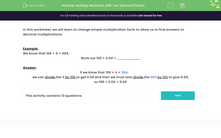# Multiply Decimals Using Multiplication Facts

In this worksheet, students will practise multiplication of decimals by using given multiplication facts.Key stage:  KS 2

Curriculum topic:   Number: Fractions, Decimals and Percentages

Curriculum subtopic:   Multiply with Two Decimal Places

Difficulty level:#### Worksheet Overview

In this worksheet, we will learn to use simple multiplication facts to allow us to find answers to decimal multiplications.

Example:

We can easily work out that 166 × 4 = 664

Work out 166 x 0.04

We know that 166 × 4 = 664

We can divide 4 by 100 to get 0.04, so we must also divide the 664 by 100 to give 6.64

So 166 × 0.04 = 6.64

Example:

We can easily work out that 259 x 3 = 777

We can use that fact to find out 259 x 0.3

We know that 259 x 3 = 777

We can divide 3 by 10 to get 0.3, so we must divide 777 by 10 to give 77.7

So 259 x 0.3 = 77.7

Count how many decimal places there are in both the calculation and the answer. There should be the same number of decimal places in both.

E.g. 0.004 x 53 has three decimal places, so the answer must have three decimal places too: 0.212.  It does!

Now it's your turn to have a go.

### What is EdPlace?

We're your National Curriculum aligned online education content provider helping each child succeed in English, maths and science from year 1 to GCSE. With an EdPlace account you’ll be able to track and measure progress, helping each child achieve their best. We build confidence and attainment by personalising each child’s learning at a level that suits them.

Get started••••